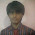Support us .Net Basics C# SQL ASP.NET ADO.NET MVC Slides C# Programs Subscribe Buy DVD

### JavaScript Basics

Suggested Videos
Part 5 - Tools for learning JavaScript
Part 6 - Inline vs external javascript
Part 7 - Where should the script tag be placed in html

In this video we will discuss
1. Is JavaScript case sensitive
3. Data types in JavaScript

Is JavaScript case sensitive
Yes, JavaScript is case sensitive programming language. Variable names, keywords, methods, object properties and event handlers all are case sensitive.

Example 1 : alert() function name should be all small letters
<script>
</script>

Example 2 : Alert() is not same as alert(). Throws Alert is not defined error. To see the error press F12 key.
<script>
</script>

Comments in JavaScript : There are 2 types of comments in JavaScript.
1) Single Line Comment

Example :
<script>
// This is a sinle line comment
</script>

2) Multi Line Comment

Example:
<script>
/* This is a
multi line
comment */
</script>

Data types in JavaScript

The following are the different data types in JavaScript
Numbers - 5, 5.234
Boolean - true / false
String - "MyString", 'MyString'

To create a variable in JavaScript use var keyword. Variable names are case sensitive.

In c# to create an integer variable we use int keyword
int X = 10;

to create a string variable we use string keyword
string str = "Hello"

With JavaScript we always use var keyword to create any type of variable. Based on the value assigned the type of the variable is inferred.
var a = 10;
var b = "MyString";

In C#, you cannot assign a string value to an integer variable
int X = 10;
X = "Hello"; // Compiler error

JavaScript is a dynamically typed language. This means JavaScript data types are converted automatically as needed during script execution. Notice that, in myVariable we are first storing a number and then a string later.
<script>
var myVariable = 100;
myVariable = "Assigning a string value";
</script>

When a + operator is used with 2 numbers, JavaScripts adds those numbers.
<script>
var a = 10;
var b = 20;
var c = a + b;
</script>

Output : 30

When a + operator is used with 2 strings, JavaScript concatenates those 2 strings
<script>
var a = "Hello "
var b = "JavaScript";
var c = a + b;
</script>

Output : Hello JavaScript

When a + operator is used with a string and a number, JavaScript converts the numeric value to a string and performs concatenation.
<script>
var a = "Number is : "
var b = 10;
var c = a + b;
</script>

Output : Number is 10

<script>
var a = "50"
var b = 10;
var c = a + b;
</script>

Output : 5010

But if you use a minus operator, numeric value is not converted to string
<script>
var a = "50"
var b = 10;
var c = a - b;1.